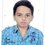NMTC Final test 2015

Hello friends, help me in finding the value of

$\large\ a = 1 + \frac { 1 }{ { 2 }^{ 2 } } + \frac { 1 }{ { 3 }^{ 2 } } + ... + \frac { 1 }{ { 2015 }^{ 2 } }$ .

Do post solution.Note by Priyanshu Mishra
3 years, 11 months ago

This discussion board is a place to discuss our Daily Challenges and the math and science related to those challenges. Explanations are more than just a solution — they should explain the steps and thinking strategies that you used to obtain the solution. Comments should further the discussion of math and science.

When posting on Brilliant:

• Use the emojis to react to an explanation, whether you're congratulating a job well done , or just really confused .
• Ask specific questions about the challenge or the steps in somebody's explanation. Well-posed questions can add a lot to the discussion, but posting "I don't understand!" doesn't help anyone.
• Try to contribute something new to the discussion, whether it is an extension, generalization or other idea related to the challenge.
• Stay on topic — we're all here to learn more about math and science, not to hear about your favorite get-rich-quick scheme or current world events.

MarkdownAppears as
*italics* or _italics_ italics
**bold** or __bold__ bold

- bulleted
- list

• bulleted
• list

1. numbered
2. list

1. numbered
2. list
Note: you must add a full line of space before and after lists for them to show up correctly
paragraph 1

paragraph 2

paragraph 1

paragraph 2

> This is a quote
This is a quote
# I indented these lines
# 4 spaces, and now they show
# up as a code block.

print "hello world"
# I indented these lines
# 4 spaces, and now they show
# up as a code block.

print "hello world"
MathAppears as
Remember to wrap math in $$...$$ or $...$ to ensure proper formatting.
2 \times 3 $2 \times 3$
2^{34} $2^{34}$
a_{i-1} $a_{i-1}$
\frac{2}{3} $\frac{2}{3}$
\sqrt{2} $\sqrt{2}$
\sum_{i=1}^3 $\sum_{i=1}^3$
\sin \theta $\sin \theta$
\boxed{123} $\boxed{123}$

Sort by:

Solution :

$\displaystyle \sum^{\infty}_{n=1}\dfrac{1}{n^{2}}=\dfrac{\pi^{2}}{6} \sim 1.64 \text{ and }\displaystyle \sum^{2015}_{n=1}\dfrac{1}{n^{2}}=a$

$\text{Simply by observation, we can conclude }\Rightarrow 1

$\text{Therefore, }\lfloor a \rfloor=\boxed{1}$

- 3 years, 11 months ago

Thank for a solution. But please explain me how you got this:

$\large\ \sum _{ n=1 }^{ \infty }{ \frac { 1 }{ { n }^{ 2 } } = \frac { \pi ^{ 2 } }{ 6 } }$ ?

- 3 years, 11 months ago

See this.

- 3 years, 11 months ago

Thanks ,now i understood. However , how many points will you give for this problem?

- 3 years, 11 months ago

75 of 400

- 3 years, 11 months ago

[a]=1

- 3 years, 11 months ago

Well,it is obvious if one knows the solution of the basel problem .However this is a much simpler question.Notice that the first term is 1,the next two terms are less than 2×1÷2^2 that is 1/2,the next four terms less than 1/4 and so on.Even if we did this til infinity,the expression would be less than 1+1/2+1/4.... which is 2.Hence the required value is 1.

- 3 years, 11 months ago

that is now we have 1+....so on .consider 1 the next terms are decreasing values in decimals .so anyway if we add also we won't get more than 1 so the integral part of that expression i.e the[a] is 1...

- 3 years, 11 months ago

Question has asked for value of a, not floor function of a. So a will be equal to approx. 1.64 . Do note that the series is going smaller and smaller.

- 3 years, 10 months ago

In the question he didn't wrote about the integral value but in the original paper it was asked to find the integer part of $a$.

- 3 years, 10 months ago

Yes, I forgot to write that.

By the way, how many questions you solved in the exam ?

- 3 years, 10 months ago

I didn't gave the exam :-(

- 3 years, 10 months ago

Ok, :) , integral value will be one only.

- 3 years, 10 months ago